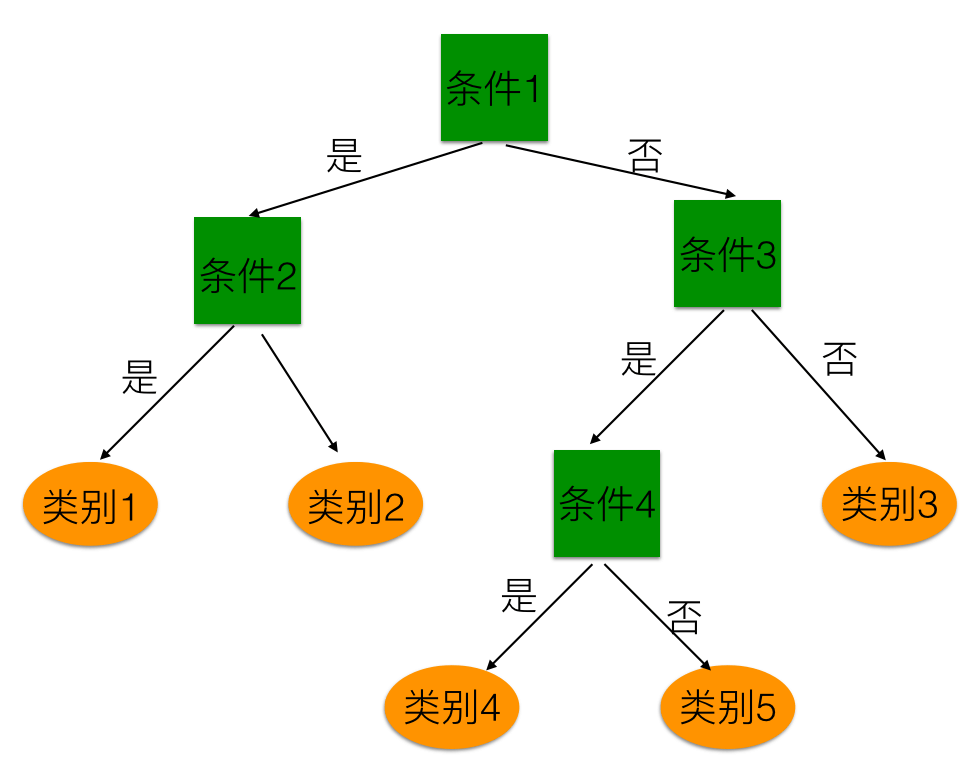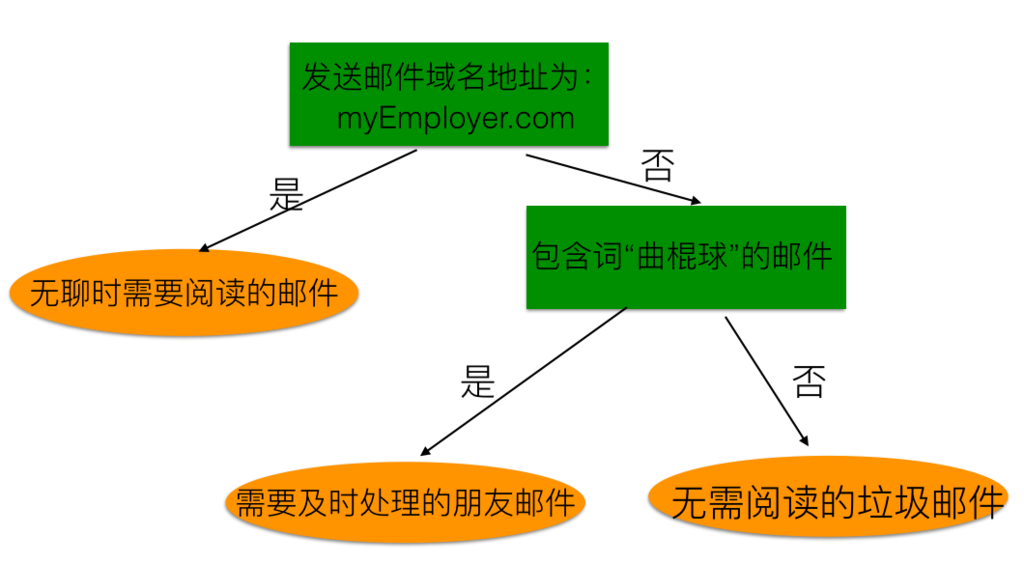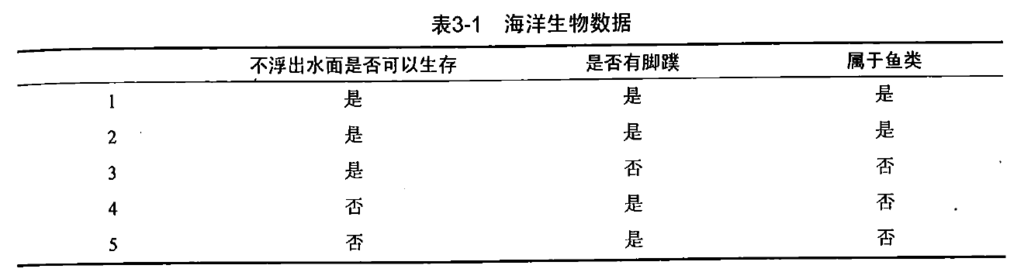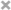## 背景

• 猜测人物，游戏的规则很简单：参与游戏的一方在脑海里想某个人，然后游戏页面一次一个选择选项陆续让你回答，比如，”这个人是在现代还是古代?”，一般问题的答案只能回答是还是不是，最后根据你一系列的回答便可以对你所想的某个人做出推断，比如你所想的这个人是你老爸。

• 投资指导或贷款申请。比如炒股，通过app新开账户后，有的会给出一个投资建议的指导，在生成这份指导建议前，会让你对某些问题(一般几个)进行回答，然后根据你的回答，生成一份对你投资类型的判断，开过这类账户的用户应该都有这个体验。另外一个就是贷款申请，会根据你的年龄、有无工作、是否有房子、信贷情况指标来决定是否给你贷款。

## 决策树模型定义## 特征选择问题### 信息增益

import numpy as np

p = np.linspace(0.,1.,11)
print p

Hp = -p*np.log2(p)-(1-p)*np.log2(1-p)
Hp = Hp[-1] = 0
print Hp

%matplotlib inline
import matplotlib
from pylab import *
import matplotlib.pyplot as plt

fig = plt.figure()
ax = fig.gca()
ax.set_xticks(p)
plt.plot(p,Hp)
grid(True)
plt.xlabel('$p$')
plt.ylabel('$H(p)$')

plt.show()


marineEnt = -0.4*np.log2(0.4)-0.6*np.log2(0.6)
print "the entropy of the marine organism: %.20f" % marineEnt
# the entropy of the marine organism: 0.97095059445466858072


def creatData():
dataSet = [[1, 1, 'yes'], [1, 1, 'yes'], [1, 0, 'no'], [0, 1, 'no'], [0, 1, 'no']]
labels = ['no surfacing', 'flippers']
return dataSet, labels

myData, labels = creatData()
print myData
# [[1, 1, 'yes'], [1, 1, 'yes'], [1, 0, 'no'], [0, 1, 'no'], [0, 1, 'no']]

from math import log

def calShannonEnt(dataSet):
dataSet = myData
numEntries = len(dataSet)
print "the number of marine organism: %d" % numEntries
labelCounts = {}
for featVec in dataSet:
currentLabel = featVec[-1]  #获取当前样本的类别标签，即"yes"或“no”
if currentLabel not in labelCounts.keys():
labelCounts[currentLabel] = 0  #如果当前样本的类别在labelCounts没有记录，则常见该类别的记录
labelCounts[currentLabel] += 1
print "label counts:"
print labelCounts
shannonEnt = 0.0
for key in labelCounts:
prob = float(labelCounts[key])/numEntries
shannonEnt -= prob*math.log(prob, 2.0)
return shannonEnt

print "the entropy of the marine organism: %.20f" % calShannonEnt(myData)

#the number of marine organism: 5
#label counts:
#{'yes': 2, 'no': 3}
#the entropy of the marine organism: 0.97095059445466858072


### 信息增益

gA1 = HD+0.4*np.log2(2.0/3.0)+0.2*np.log2(1.0/3.0)
print "g(D, A1): %f" %gA1
gA2 = HD-0.8
print "g(D, A2): %f" %gA2
#g(D, A1): 0.419973
#g(D, A2): 0.170951


1 1 1 yes
2 1 1 yes
3 1 0 no
4 0 1 no
5 0 1 no

def splitDataSet(dataSet, axis, value):
retDataSet = []
for featVec in dataSet:
if featVec[axis] == value:
reducedFeatVec = featVec[:axis]
reducedFeatVec.extend(featVec[axis+1:])
retDataSet.append(reducedFeatVec)
return retDataSet

dataSet = myData
axis = 0
value = 1
myDat = splitDataSet(dataSet, axis, value)
print myDat
#myDat的输出：[[1, 'yes'], [1, 'yes'], [0, 'no']]


numFeatures = len(dataSet) - 1
baseEntropy = calShannonEnt(dataSet)
print "Base entropy: %f" % baseEntropy

print

bestInGain = 0.0
bestFeature = -1
for i in range(numFeatures):
featList = [example[i] for example in dataSet]
print "==========select feature========="
print featList
print
uniqueVals = set(featList)
newEntropy = 0.0
for value in uniqueVals:
subDataSet = splitDataSet(dataSet, i, value)
print "sub-dataset: "
print subDataSet
print
prob = len(subDataSet)/float(len(dataSet))
newEntropy += prob*calShannonEnt(subDataSet)
infoGain = baseEntropy - newEntropy
print "information gain: %f20" % infoGain
if (infoGain > bestInGain):
bestInGain = infoGain
bestFeature = i

print "\nThe selected best feature: %d" % bestFeature


the number of marine organism: 5
label counts:
{'yes': 2, 'no': 3}
Base entropy: 0.970951

==========feature candidate=========
[1, 1, 1, 0, 0]

sub-dataset:
[[1, 'no'], [1, 'no']]

the number of marine organism: 2
label counts:
{'no': 2}
sub-dataset:
[[1, 'yes'], [1, 'yes'], [0, 'no']]

the number of marine organism: 3
label counts:
{'yes': 2, 'no': 1}
information gain: 0.41997320
==========feature candidate=========
[1, 1, 0, 1, 1]

sub-dataset:
[[1, 'no']]

the number of marine organism: 1
label counts:
{'no': 1}
sub-dataset:
[[1, 'yes'], [1, 'yes'], [0, 'no'], [0, 'no']]

the number of marine organism: 4
label counts:
{'yes': 2, 'no': 2}
information gain: 0.17095120

The selected best feature: 0


### 信息增益比